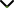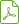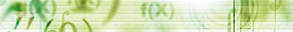Qualitative Theory of Differential, Difference, and Dynamic Equations

Publishing date
04 Apr 2014
Status
Published
15 Nov 2013

Guest Editors

1School of Control Science and Engineering, Shandong University, Jinan, Shandong 250061, China

2Department of Mathematics, Faculty of Art and Science, Nigde University, 51200 Nigde, Turkey

3Ramanujan Institute for Advanced Study in Mathematics, University of Madras, Chennai 600 005, India

Qualitative Theory of Differential, Difference, and Dynamic Equations

DescriptionThis special issue is mainly devoted to a rapidly developing branch of the qualitative theory of (partial) differential equations, difference equations, and dynamic equations on time scales with or without deviating arguments. It covers oscillation and asymptotic theories and stability theory of previously said equations and their systems, as well as their applications in numerical analysis, mathematical biology, economics and finance, and other related areas. Research on oscillation/nonoscillation of solutions of these equations is currently very active, and this special issue will contain high-quality papers on current related topics written by world-leading experts in the area. Potential topics include, but are not limited to:

• Oscillation/nonoscillation theory for differential equations, difference equations, and dynamic equations on time scales
• Asymptotic behavior of solutions of differential equations, difference equations, and dynamic equations on time scales
• Stability theory of differential equations, difference equations, and dynamic equations on time scales
• Applications to numerical analysis, mathematical biology, economics and finance, and other sciences
• Considered equations may be of first-order equations, higher order equations, systems of equations, Sturm-Liouville equations, and Hamiltonian systems

Before submission authors should carefully read over the journal’ Author Guidelines, which are located at http://www.hindawi.com/journals/ijde/guidelines/. Prospective authors should submit an electronic copy of their complete manuscript through the journal Manuscript Tracking System at http://mts.hindawi.com/submit/journals/ijde/odd/ according to the following timetable:Download Special IssueJournal metrics
Acceptance rate25%
Submission to final decision46 days
Acceptance to publication47 days
CiteScore1.900
Journal Citation Indicator0.520
Impact Factor-Author guidelinesEditorial boardDatabases and indexing

Article of the Year Award: Outstanding research contributions of 2020, as selected by our Chief Editors. Read the winning articles.